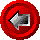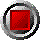Variable Principal Swaptions (Black-76 model)Variable Principal Swaptions (Black-76 model)

Swaption_Black_Var(argument list…)

This function values European swaptions on variable principal structures. The calling functions allow the user to choose between three different types of swaptions using a Swaption_Type alphabetic switch:

 R receiver swaption P payer swaption S straddle (combination of payer + receiver)

The calling functions allow the user to choose from six different calculations, indexed by an alphabetic Output_Type switch:

 P option price D delta (derivative with respect to forward yield) G gamma (second derivative with respect to forward yield) V vega (derivative with respect to volatility, 1% change) T theta (time decay over next day) I implied yield volatility

An Output_Type/Swaption_Type may be entered in either upper or lower case, and the user many substitute the entire word for the first letter if so desired (only the first letter is scanned). Delta and gamma are returned in terms of swaps (i.e. an at the money swaption would have a delta of approximately +/-0.5). The price, vega, and theta are in terms of percentage of the notional of the swap.

The functions use the following arguments:

 Argument Description Restrictions Swaption_Type type of swaption R, P or S (see above) Output_Type output returned by function P, D, G, V, T or I (see above) Valuation_Date valuation date (e.g. today) valid Excel date number Swaption_Maturity_Date expiry date of the swaption valid Excel date number >= valuation date Swap_Start_Date start date of swap valid Excel date numbers >= Swaption_Maturity_Date Prin_Dates array of Excel date numbers indicating notional swap principal dates valid Excel date numbers >= Valuation_Date, in strictly ascending order The first element of this array must be Swap_Start_Date. The last element must be the maturity date of the underlying swap. Prin_Amounts array of notional swap principal amounts > 0, corresponding to Principal_Dates array (note that the last element in this array is not used by the function) Year_Basis year basis used in determining payments 360 or 365 Par_Swap_Rate current fair fixed swap rate in decimal form (e.g. six percent entered as 0.06) [see FwdParSwapRateVarPrin function] > 0 Strike_Rate strike rate of the swaption in decimal form > 0 Zero_Dates array of zero coupon curve dates strictly ascending order The first date of this array must be Valuation_Date Zero_Rates array of continuously compounded riskless rates in decimal form (e.g. six percent entered as 0.06) corresponding to Zero_Dates > 0 Yield_Vol or Opt_Price underlying swap rate volatility in decimal form, or market price of the swaption (when Output_Type = I) > 0

© 1995-98 Leap of Faith Research Inc.